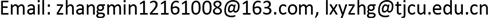AAMAdvances in Applied Mathematics2324-7991Scientific Research Publishing10.12677/AAM.2018.77093AAM-25947AAM20180700000_72449481.pdf数学与物理 动态价格下Gompertz系统的捕捞问题 Harvesting in Gompertz System with Dynamic Price 21广31天津商业大学理学院，天津天津商业大学经济学院，天津null090720180707776781© Copyright 2014 by authors and Scientific Research Publishing Inc. 2014This work is licensed under the Creative Commons Attribution International License (CC BY). http://creativecommons.org/licenses/by/4.0/

1天津商业大学经济学院，天津

2天津商业大学理学院，天津Copyright © 2018 by authors and Hans Publishers Inc.1. 引言

{ d s d t = F ( s ) − E s , d E d t = v ( p E s − w E ) , (1)

p ( S ) = n m + S ,

lim S → 0 p ( S ) = n m = p max ,   lim S → ∞ p ( S ) = 0 ,

{ d s d t = F ( s ) − α E s , d E d t = v α E s ( n m + α E s − w α s ) , (2)

d p d t = β ( q d − q s ) , β > 0. (3)

d p d t = β ( a − c ) + β ( b − d ) p . (4)

{ d s d t = r s ln ( k / s ) − α E s , d E d t = v π = v ( p α E s − w E ) , d p d t = β ( a − c ) + β ( b − d ) p , (5)

2. 平衡点及其稳定性分析

{ r s ln ( k / s ) − α E s = 0 , p α E s − w E = 0 , β ( a − c ) + β ( b − d ) = 0 ,

E * = ( s * , E * , p * ) .

s * = w p * α ， E * = r α ln ( k / s * ) ， p * = a − c d − b

J = ( r ln ( k / s ) − r − α E − α s 0 v p α E v ( p α s − w ) v α E s 0 0 β ( b − d ) )

a 0 λ 3 + a 1 λ 2 + a 2 λ + a 3 = 0 ,

a 0 = 1 , a 1 = r − β ( b − d )

a 2 = [ v w r ln ( k / s * ) − r β ( b − d ) ]

a 3 = − β ( b − d ) v w r ln ( k / s * )

Δ 2 = [ r − β ( b − d ) ] [ v w r ln ( k / s * ) − r β ( b − d ) ] + β ( b − d ) v w r ln ( k / s * ) = r [ v w r ln ( k / s * ) − r β ( b − d ) ] + r β 2 ( b − d ) 2

3. 开放渔业的动力学行为

4. 小结

Increasing or decreasing of population and effort in four quadrant
sEsE
III
IIIIV